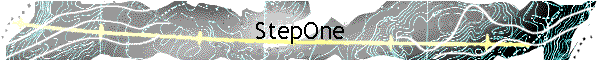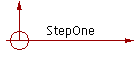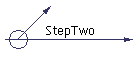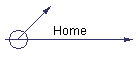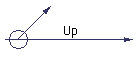To decrypt the Riddle, first lay out the words so they can be read and analyzed. (Suggest you click on the thumbnail below to see the original large image, then check and print out the table below to use as a worksheet - pencil and paper- intuitive trial and error are key to breaking any code. It can be tedious - in fact, I wouldn't be surprised if I made a mistake somewhere in the encoding-decoding sequence - I am not a very meticulous person - never could have worked at Bletchley Park!)

Of course, an authentic tablet from the time of Christ would likely be in Aramaic or Greek, not English - but we have to work with what we have.

From the engraved tablet: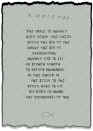to letters on the worksheet:

 y m j n r f l j t k h m w n x y q n j x g j m n i y m j l w j j s b f y j w y m j b f d y t y m j q n e m y y m j b f d y t j a j u g f x y n s l h m w n x y x t r t k l t i f s I k f y m j w x f a n t w t k h f j x f w u m n q n u u n n s y m j x m f I t b t k y m j r t z s y t k r t x j x q t a j t k l t i r f s b t r f s f s i h m n q i f w j u w t h q f d r j i y t f q q